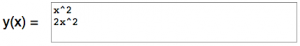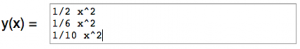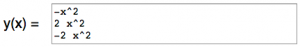# Let's Get Started

Given verbal, graphical, or symbolic descriptions of the graph of y = ax2 + c,  the student will investigate, describe, and predict the effects on the graph when "a" is changed.

TEKS Standards and Student Expectations

A(7) Quadratic functions and equations. The student applies the mathematical process standards when using graphs of quadratic functions and their related transformations to represent in multiple ways and determine, with and without technology, the solutions to equations. The student is expected to:

A(7)(C) determine the effects on the graph of the parent function f(x) = x2 when f(x) is replaced by af(x), f(x) + d, f(x – c), f(bx) for specific values of a, b, c, and d

Resource Objective(s)

Determine the effect of changing the "a" value on the graph of y = ax2 + c

Essential Questions

How is the parabola affected if "a" has a value greater than 1 in the equation y = ax2?

How is the parabola affected if "a" has a value between 0 and 1 in the equation y = ax2 + c?

How is the parabola affected if "a" has a value less than 1 in the equation y = ax2 + c?

Vocabulary

# Analyzing the Effect When a > 1

Let's look at how changing the value of "a" to a number greater than 1 will affect the graph of y = ax2.

Use the applet below or click on the link to access an online graphing calculator.

Directions:
You will be entering quadratic equations in the y(x) field. (Remember, this is function notation. It is just a different way of writing a "y =" equation.) To enter the exponent of the equation y(x) = x^2, use the ^ symbol which is accessed by pressing "Shift 6" on your keyboard. The equation will be written in the y(x) field as x^2. At any time, click on "Show Tabular Data" to see a table of values.

1. Enter x^2 in the y(x) field and then click on "Plot/Update" to graph the equation.

2. Go to the next line in the y(x) field and enter 2x^2. Then click on "Plot/Update." A snapshot of your entries is shown.3. Go to the next line in the y(x) field and enter 5x^2. Then click on "Plot/Update."

4. In your notes, describe how changing the coefficient of x from 1 to a number greater than 1 affects the graph. (Use the words "narrower" or "wider".) Sketch the graphs of y = x^2 and y = 5x^2 to illustrate your findings.

# Analyzing the Effect When 0 < a < 1

Now let's look at what happens to the graph of y = ax2 when the value of "a" is between 0 and 1.

Use the applet below, or click on the link to access an online graphing calculator.

Directions:

1. Enter x^2 in the y(x) field. Click on "Plot/Update" to graph the equation.

2. Go to the next line in the y(x) field and enter 1/2x^2. Then click on "Plot/Update." (You can enter the fraction by using the "/" key on your keyboard.)

3. Go to the 3rd line in the y(x) field and enter 1/6x^2. Then click on "Plot/Update."

4. Go to the 4th line in the y(x) field and enter 1/10x^2. Then click on "Plot/Update."

A snapshot of your entries is shown here:5. In your notes, describe how changing the coefficient of x from 1 to a number greater than 0 but less than 1 affects the graph. (Use the words "narrower" or "wider".)

# Analyzing the Effect When a < 0

Finally, we are going to explore how the graph of y = ax2 is affected when the value of "a" is less than 0.

Use the applet below or click on the link to access an online graphing calculator.

Directions:

1. Enter x^2 in the y(x) field and click on "Plot/Update" to graph the equation.

2. Go to the next line in the y(x) field and enter -x^2. Then click on "Plot/Update."

3. Go to the 3rd line in the y(x) field and enter 2x^2. Then click on "Plot/Update."

4. Go to the 4th line in the y(x) field and enter -2x^2. Then click on "Plot/Update."

A snapshot of your entries is shown.5. Go to the 5th line in the y(x) field and enter 1/3x^2. Then click on "Plot/Update." (You can enter the fraction by using the "/" key on your keyboard.)

6. Go to the 6th line in the y(x) field and enter -1/3x^2. Then click on "Plot/Update."

7. In your notes, describe how changing the coefficient of x from 1 to a number less than 0 affects the graph. (Use the words "narrower" or "wider".)